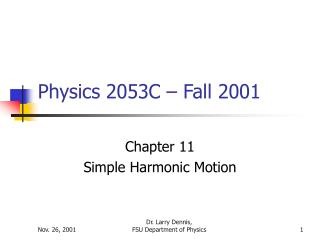Download PresentationPhysics 2053C – Fall 2001

# Physics 2053C – Fall 2001 - PowerPoint PPT PresentationDownload Presentation## Physics 2053C – Fall 2001

- - - - - - - - - - - - - - - - - - - - - - - - - - - E N D - - - - - - - - - - - - - - - - - - - - - - - - - - -
##### Presentation Transcript

1. Physics 2053C – Fall 2001 Chapter 11 Simple Harmonic Motion Dr. Larry Dennis, FSU Department of Physics

2. TH QH W QL Engine TL Carnot Cycle  The most efficient Engine Possible • Q = U + W •  = W/Qin = (QH-QL)/(QH) = (TH-TL)/(TH)  = 1 – (TL/TH )

3. TH QH W QL Engine TL Carnot Cycle  The most efficient Engine Possible Example: An engine absorbs 200 J of heat and does 75 J of work per cycle. What is it’s efficiency? How much heat does it eject into the cold reservoir? If the temperature of the cold reservoir is 190 K, what is the temperature of the hot reservoir? If the engine undergoes 2500 cycles per second, what is it’s power?

4. TH QH W QL Engine TL Carnot Cycle  The most efficient Engine Possible What is it’s efficiency?  = Work/Heat In = 75 J/200 J = .375 How much heat does it eject into the cold reservoir? Q = U + W QH – QL = U + W QL = QH – W = 200 – 75 J = 125 J

5. TH QH W QL Engine TL Carnot Cycle  The most efficient Engine Possible If the temperature of the cold reservoir is 190 K, what is the temperature of the hot reservoir? = 1 – TL/TH = 0.375 TH – TL = 0.375 * TH TL = TH – 0.375 * TH TL = 0.625*TH TH = TL/0.625 = 190 / 0.625 = 304 K

6. TH QH W QL Engine TL Carnot Cycle  The most efficient Engine Possible If the engine undergoes 2500 cycles per second, what is it’s power? P = W/t = 2500 * 75 J/1 s P = 187500 Watts Note this is about 250 h.p.

7. Periodic Motion • Many kinds of motion are periodic. • Examine within the context of: • Forces • Energy • Equilibrium Position & Restoring Forces

8. Position vs. Time x = A sin(2*t/T) Position = Amplitude * sin( 2 * time/Period) x Amplitude = A t Period = T

9.  = 2f = 2/T = (k/m) Spring Oscillations x k m x(t) = Acos(t) or Asin(t)

10. T Net Force mg  = 2f = 2/T = (g/L) Pendulum Oscillations L (t) = A cos(t) or (t) = A sin(t) Note: This does not depend on the mass!!

11. max Energy in Simple Harmonic Motion • As expected Energy is conserved. • Oscillates between potential and kinetic energy. All Potential Energy All Kinetic Energy min

12. Total Energy Kinetic Energy Potential Energy Energy for springs. • As expected Energy is conserved. • Oscillates between potential and kinetic energy. PE = ½kx(t)2 = ½kA2cos2(t) KE = ½mv(t)2 = ½m2A2sin2(t) KEmax = ½m2A2 = PEmax = ½kA2 Note: 2 = k/m

13. CAPA 6-10 • A 368g mass vibrates according to the equation x = 0.346 sin (5.20 t) where x is in meters and t is in seconds. Determine the amplitude. • Determine the frequency. • Determine the period. • Determine the total energy. • Determine the kinetic energy when x is 12.5 cm. x = 0.346 sin (5.20 t) Compare this with the standard formula: x = A sin (t) A = 0.346 m and  = 5.20 rad/s

14. CAPA 6 • A 368g mass vibrates according to the equation x = 0.346 sin (5.20 t) where x is in meters and t is in seconds. Determine the amplitude. x = 0.346 sin (5.20 t) Compare this with the standard formula: x = A sin (t) A = 0.346 m and  = 5.20 rad/s Amplitude = 0.346 m

15. CAPA 7 A 368g mass vibrates according to the equation x = 0.346 sin (5.20 t) where x is in meters and t is in seconds. • Determine the frequency. x = 0.346 sin (5.20 t) Compare this with the standard formula: x = A sin (t) A = 0.346 m and  = 5.20 rad/s 2f =   f = /2 = 5.20/(2*3.14159) = 0.828 s-1

16. CAPA 8 A 368g mass vibrates according to the equation x = 0.346 sin (5.20 t) where x is in meters and t is in seconds. • Determine the period. T = 1/f  T = 1/0.828 s-1 = 1.21 s

17. CAPA 9 A 368g mass vibrates according to the equation x = 0.346 sin (5.20 t) where x is in meters and t is in seconds. • Determine the total energy. Total Energy = ½ kA2 2 = k/m  k = m2 = 0.368 * 5.22 = 9.95 N/m Total Energy = ½ * 9.95 N/m * (.346 m)2 Total Energy = 0.596 J

18. CAPA 10 A 368g mass vibrates according to the equation x = 0.346 sin (5.20 t) where x is in meters and t is in seconds. • Determine the kinetic energy when x is 12.5 cm KE = Total Energy – Potential Energy KE = .596 – ½kx2 KE = .596 – ½*9.95 *.1252 KE = .518 J

19. Next Time • Quiz on Chapter 15 • First Law • Engines and Efficiency. • Please see me with any questions or comments. See you on Wednesday.